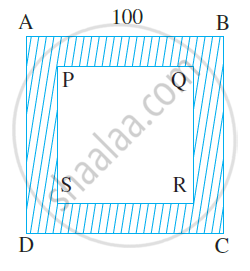# A path 5 m wide runs along inside a square park of side 100 m. Find the area of the path. Also, find the cost of cementing it at the rate of Rs. 250 per 10 m2. - Mathematics

Sum

A path 5 m wide runs along inside a square park of side 100 m. Find the area of the path. Also, find the cost of cementing it at the rate of Rs. 250 per 10 m2.

#### SolutionLet ABCD be the square park of side 100 m. The shaded region represents the path 5 m wide.

PQ = 100 – (5 + 5) = 90 m

Area of square ABCD = (side)2 = (100)2 m2 = 10000 m2

Area of square PQRS = (side)2 = (90)2 m2 = 8100 m2.

Therefore, area of the path = (10000 − 8100) m2 = 1900 m2

Cost of cementing 10 m2 =Rs. 250

Therefore, cost of cementing 1 m2 = Rs. 250/10

So, cost of cementing 1900 m2 = Rs. 250/10 xx 1900 = Rs. 47,500.

Is there an error in this question or solution?
Share